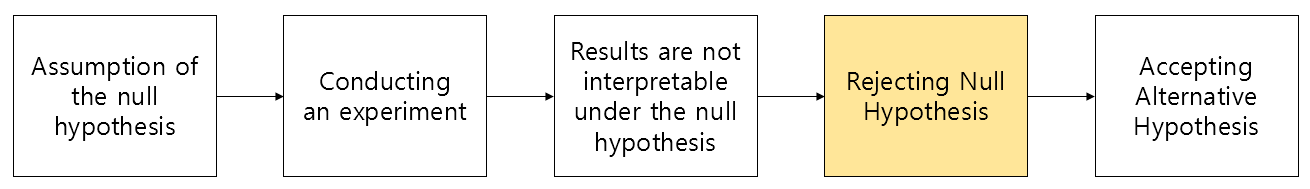# The Theory of Praying for Failure - Null Hypothesis and Alternative Hypothesis.

Regardless of the field, one of the difficulties encountered when studying something new is the terminology.

Therefore, many times studying the field becomes clearer only after becoming familiar with the terminology.

If we were to pick difficult terms encountered in statistics, hypotheses would be one of them.

Null hypothesis and alternative hypothesis are representative terms, but they are very unfriendly terms that do not provide any help even when translated into English (they can be translated as null hypothesis and alternative hypothesis, respectively).

In my case, I studied statistics on my own in graduate school, and it took over a year to become familiar with these terms.

In this article, let’s explore the meanings and reasons why these concepts are necessary by looking at the terms related to these hypotheses.

# Null Hypothesis and Alternative Hypothesis

We want to prove that we have discovered a new fact through research/development.

At this time, null hypothesis and alternative hypothesis are used, which can be thought of as hypotheses of “there is nothing new” and “there is something new,” respectively.

For example, the null hypothesis and alternative hypothesis would be as follows:

e.g.) If we are researching whether smoking affects the increase in brain diseases,

• Null Hypothesis: Smoking status does not affect the occurrence of cerebrovascular diseases.
• Alternative Hypothesis: Smoking status affects the occurrence of cerebrovascular diseases.

However, there is one thing that is not easily understood at a common sense level.

"Why do we need to introduce the unnecessary concept of 'null hypothesis' when we can simply use the alternative hypothesis which is the hypothesis of 'there is something new'?"

There could be several reasons why the null hypothesis is introduced, but we can roughly categorize them into three.

1. Proving that something is not true is much easier than proving that something is true.
2. Describing the null hypothesis “correctly” is less likely to fail than describing the alternative hypothesis “accurately.”
3. We cannot know the population parameter, and subjectivity should not be involved in research. Translation:

Due to various reasons, in traditional statistics, it is indirectly attempted to confirm a new hypothesis by failing to reject the null hypothesis. This method can be seen as being closely related to reductio ad absurdum1.

Reductio ad absurdum is a way of indirectly proving a proposition. Since it may be difficult to directly prove that a proposition is true, the negation of the proposition is assumed to be true, and the proof is carried out until a contradiction is found, thereby proving the original proposition.

In other words, if you want to test a hypothesis that there is a change in an investigation or research, you conduct the experiment assuming that there is no change.

Then, if you discover a contradiction in the hypothesis that there is no change, you can indirectly prove the fact that there is a change based on this evidence.

Therefore, the process of hypothesis testing using the null hypothesis can be summarized as follows:Figure 1. Hypothesis testing process using the null hypothesis

The method of testing using the null hypothesis can be likened to the “presumption of innocence” in law. According to the presumption of innocence, a suspect or defendant is presumed innocent (null hypothesis is not rejected) until proven guilty (null hypothesis is rejected and alternative hypothesis is supported).

At this point, in order to find the defendant guilty, there must be evidence or circumstances (statistically significant level) that cannot occur if the defendant is actually innocent.

## Caution on Null Hypothesis/Alternative Hypothesis

One key point to be cautious about with null hypothesis/alternative hypothesis is that rejecting the null hypothesis does not necessarily prove the alternative hypothesis.

In other words, the focus of the null hypothesis testing process is solely on “failure to reject” the null hypothesis.

This is a very important issue in the field of research, and we will discuss it in more detail when we cover p-values.

• Helmenstine, Anne Marie, Ph.D. “Null Hypothesis Definition and Examples.” ThoughtCo, Feb. 11, 2020, thoughtco.com/definition-of-null-hypothesis-and-examples-605436.
• “Doctor Bae’s Fluent Biostatistics,” by Jeong Min Bae, Hannarae Academy
• Null hypothesis, Wikipedia
• Statistical hypothesis testing, Wikipedia
1. Surprisingly, reductio ad absurdum is often used in everyday life as well. The expression “Let’s assume that what you’re saying is correct~” is an example of using reductio ad absurdum.